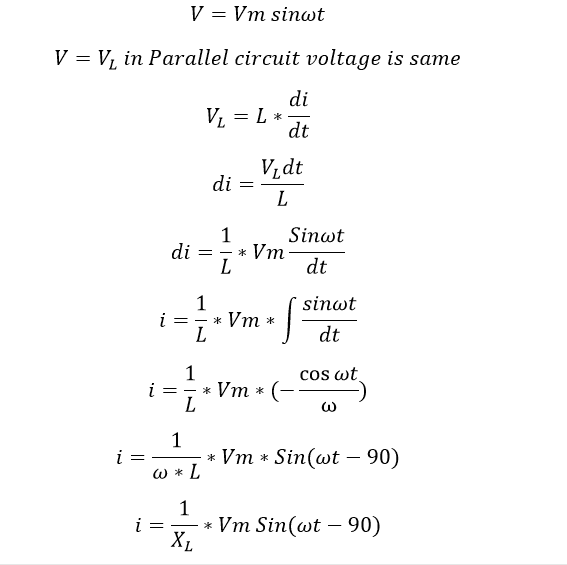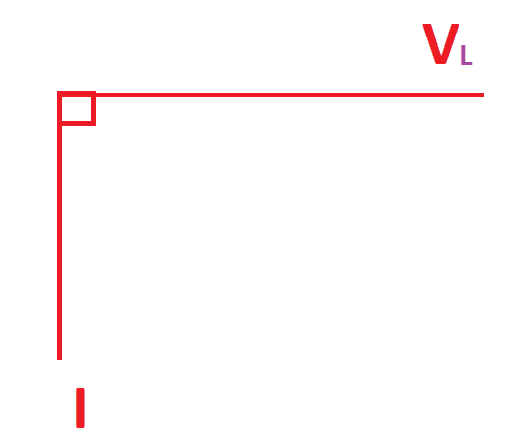# Why Inductor Has Lagging Power Factor

### Why Inductor Has Lagging Power factor:

Inductor is a one of the fundamental passive components of the electric circuit. Inductance is defined as the property of an electric circuit by virtue of which a varying current induces an electromotive force is a neighboring circuit. It is also defined as the Property of the conductor or circuit that establishes magnetic flux linkages. Or Simply inductance is a property of the inductor. In this, tutorial we are going to see why inductor lags the current always or why we are saying inductor as lagging power factor.

Let’s see the emf induced or voltage across to the inductor is V and di/dt the rate of change of current in the inductor L. hence the emf induced in the coil is equal to…From above equation. XL is called inductive reactance.

### Inductor phasor diagram:Here you see We apply a sinusoidal voltage and We get the current value of -90 deg. The negative angle says about the lags nature. Hence in a pure inductor, the inductive current always lags behind the voltage by 90deg. Let see the power flow in an inductor.